# ML Aggarwal Solutions for Class 9 Maths Chapter 6 Problems on Simultaneous Linear Equations

ML Aggarwal Solutions for Class 9 Maths Chapter 6 Problems on Simultaneous Linear Equations contains solutions to exercise problems which are created by subject matter experts at BYJU’S. Mathematics is one of the high scoring subjects for all students. Hence, the students must comprehend the concepts first and then start solving the problems. Practising these problems on a daily basis is the key to scoring high marks in examinations. Further, students can refer to solutions of this chapter from the ML Aggarwal Solutions for Class 9 Maths Chapter 6 Problems on Simultaneous Linear Equations PDF, available in the link given below.

Chapter 6 consists of problems in finding the unknown numbers, quantities, work of men/women and age of people, as per the latest ICSE guidelines. ML Aggarwal solutions are available in PDF format so that the students can use them while solving the exercise wise problems of ML Aggarwal textbook. Using this resource, students can strengthen their problem-solving skills, which are important from an examination point of view.

## ML Aggarwal Solutions for Class 9 Maths Chapter 6 Problems on Simultaneous Linear Equations Download PDF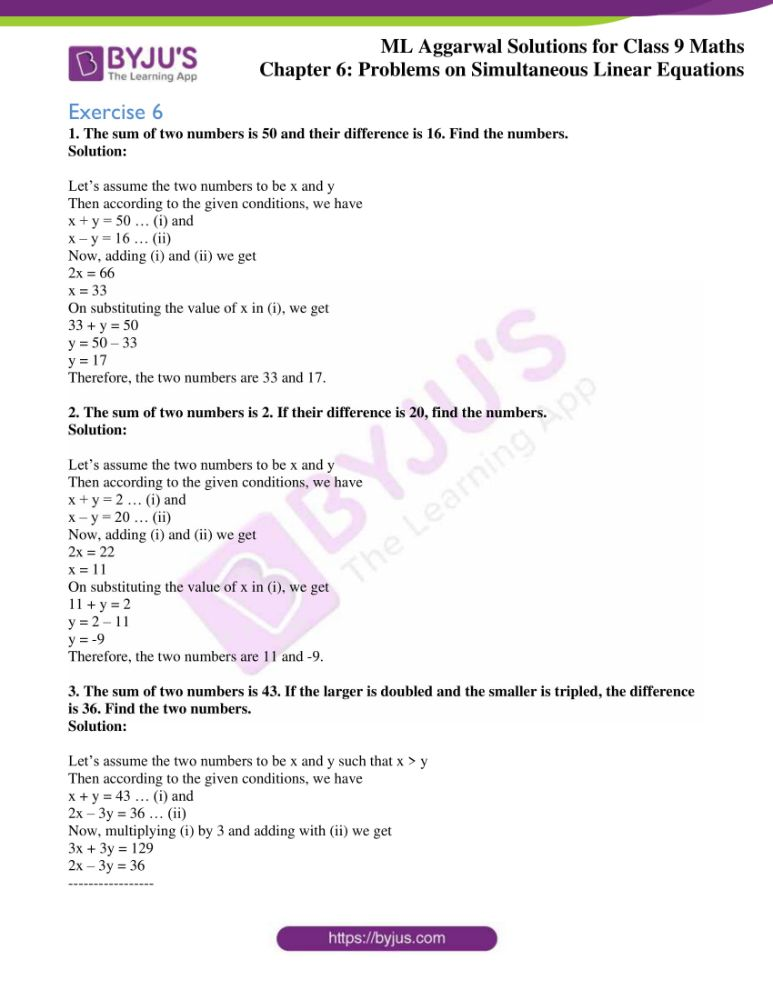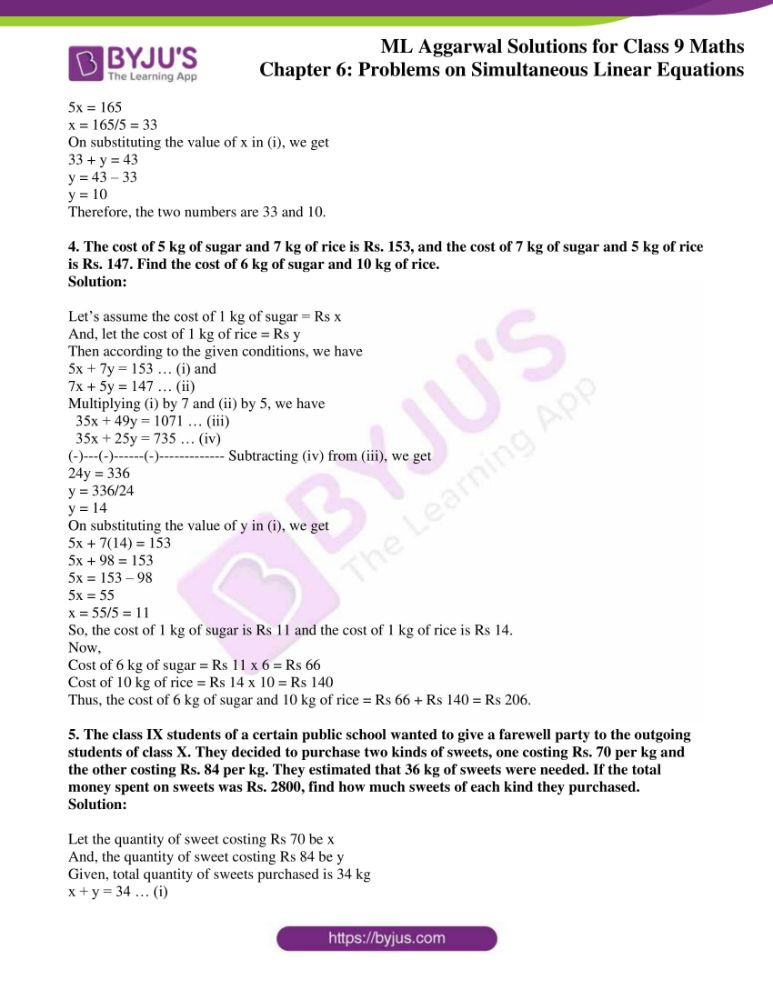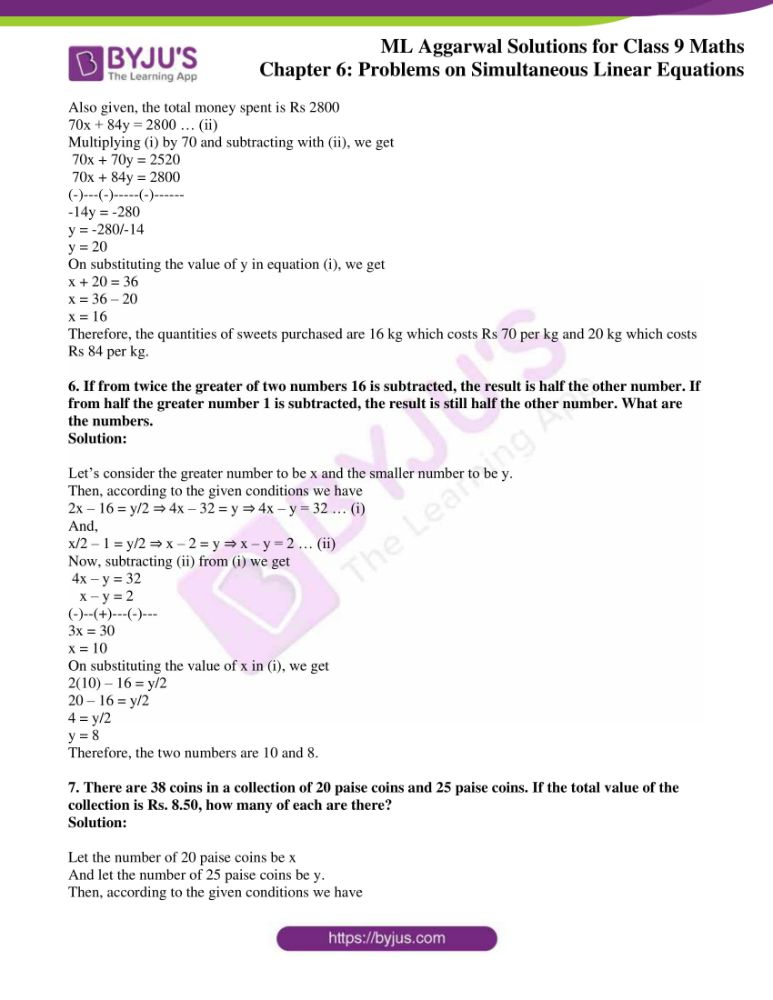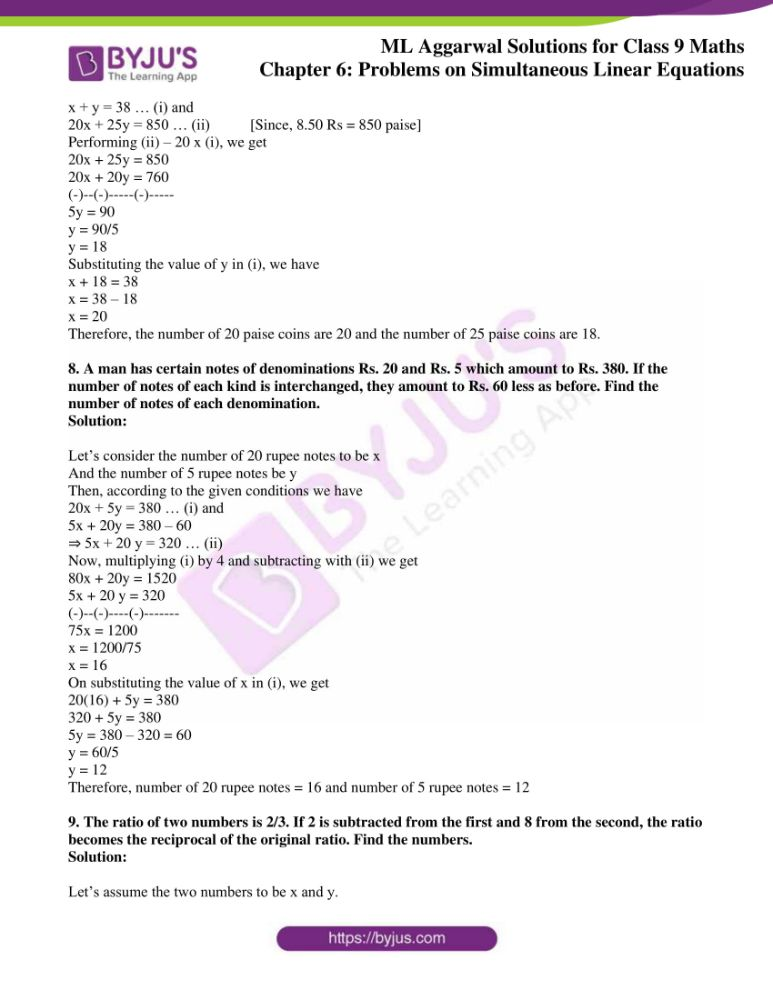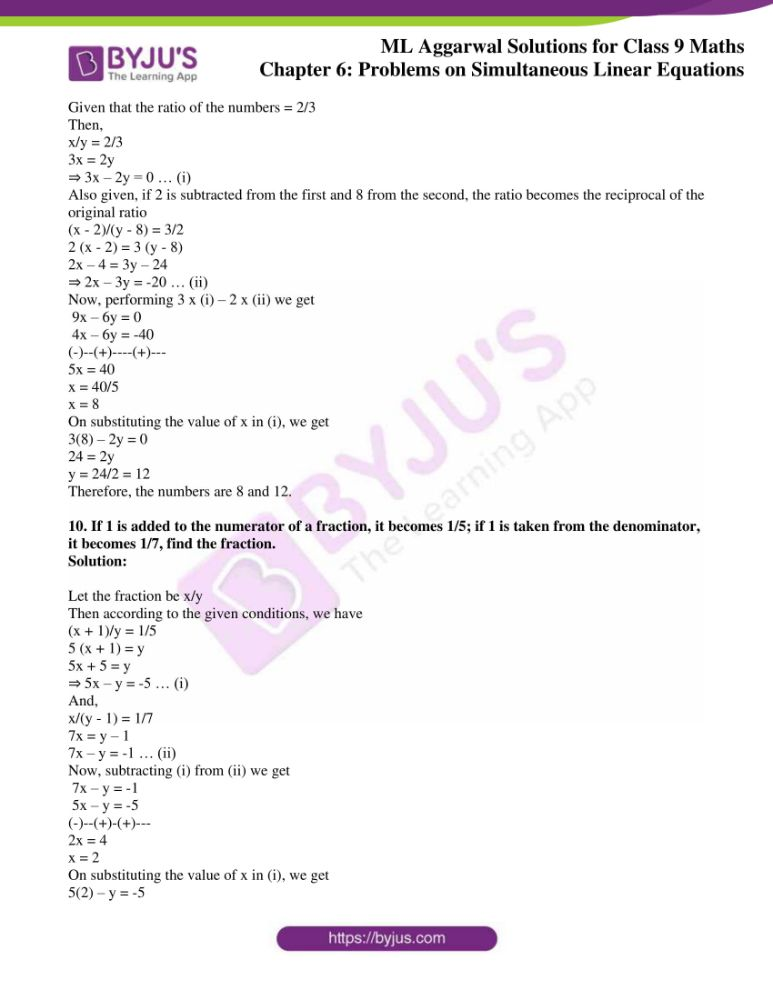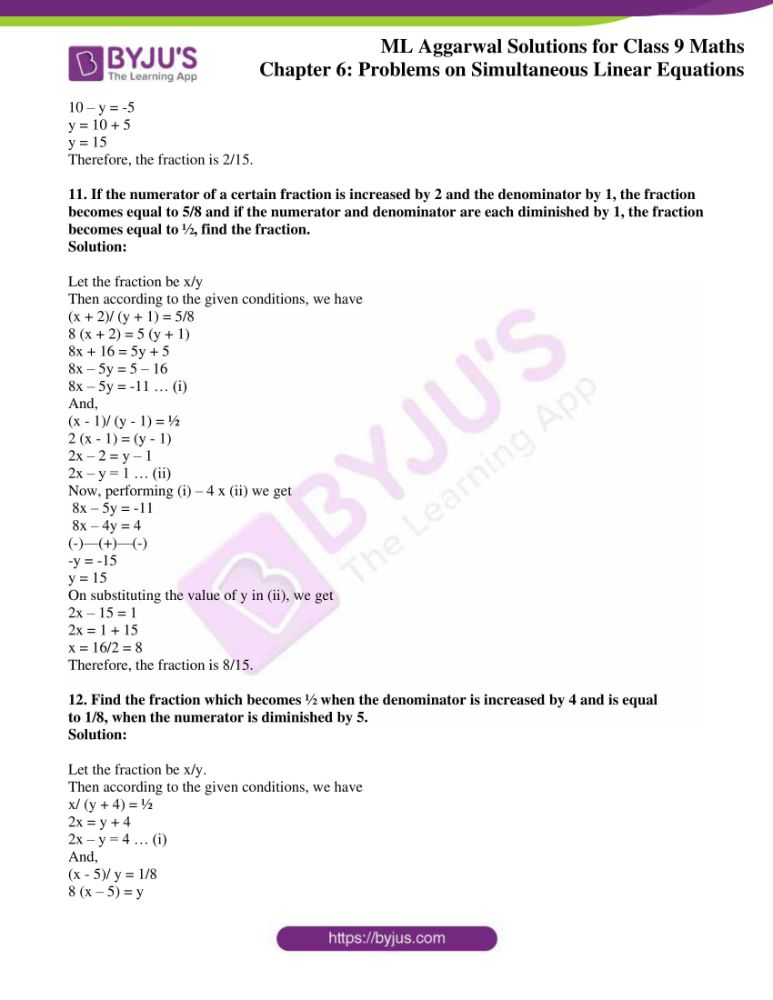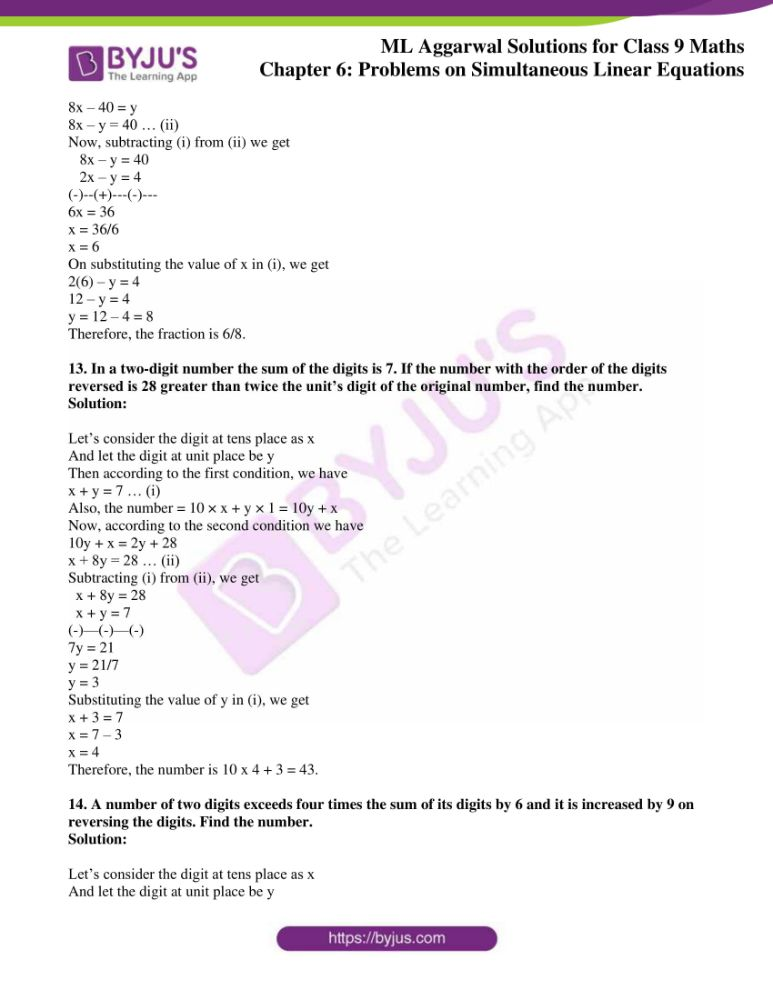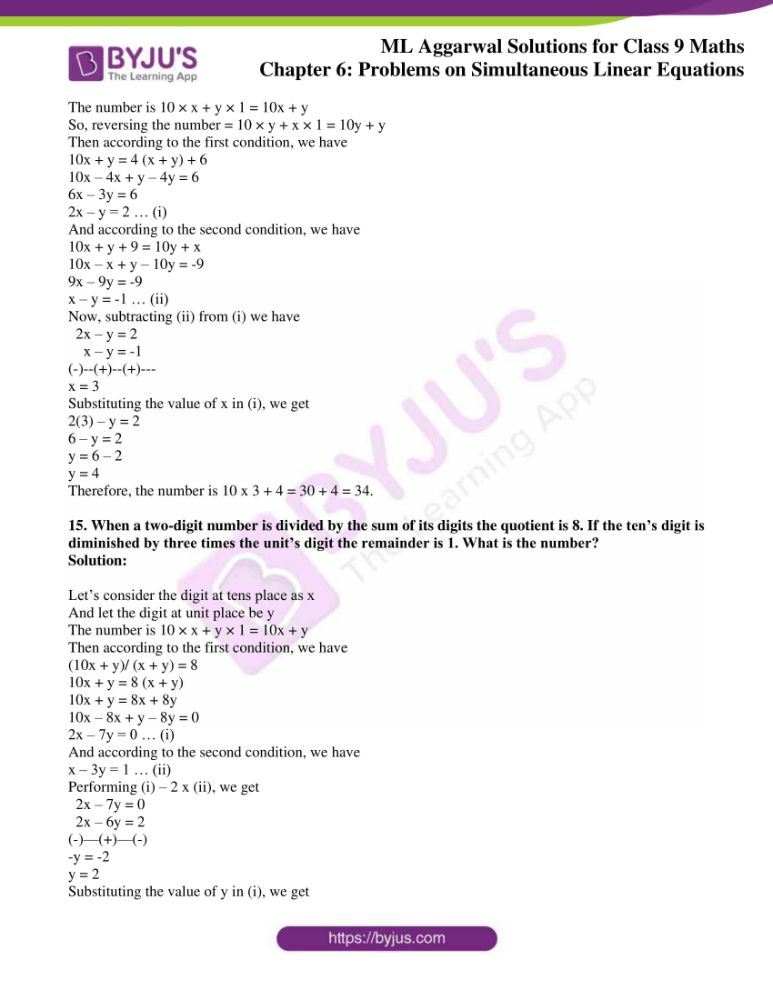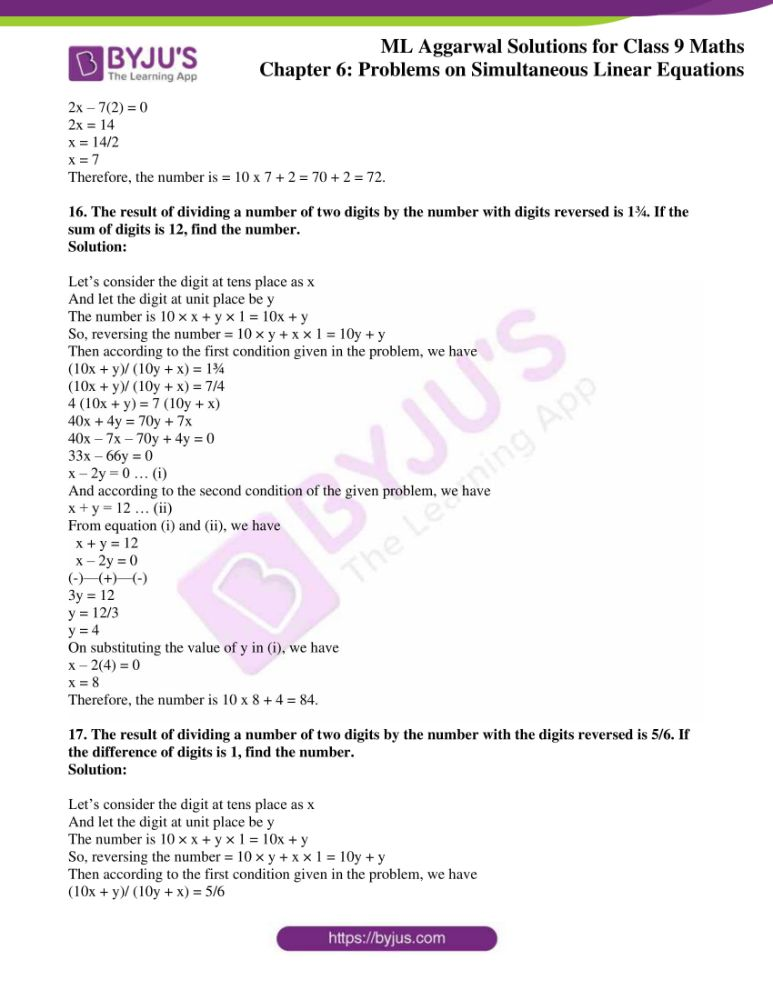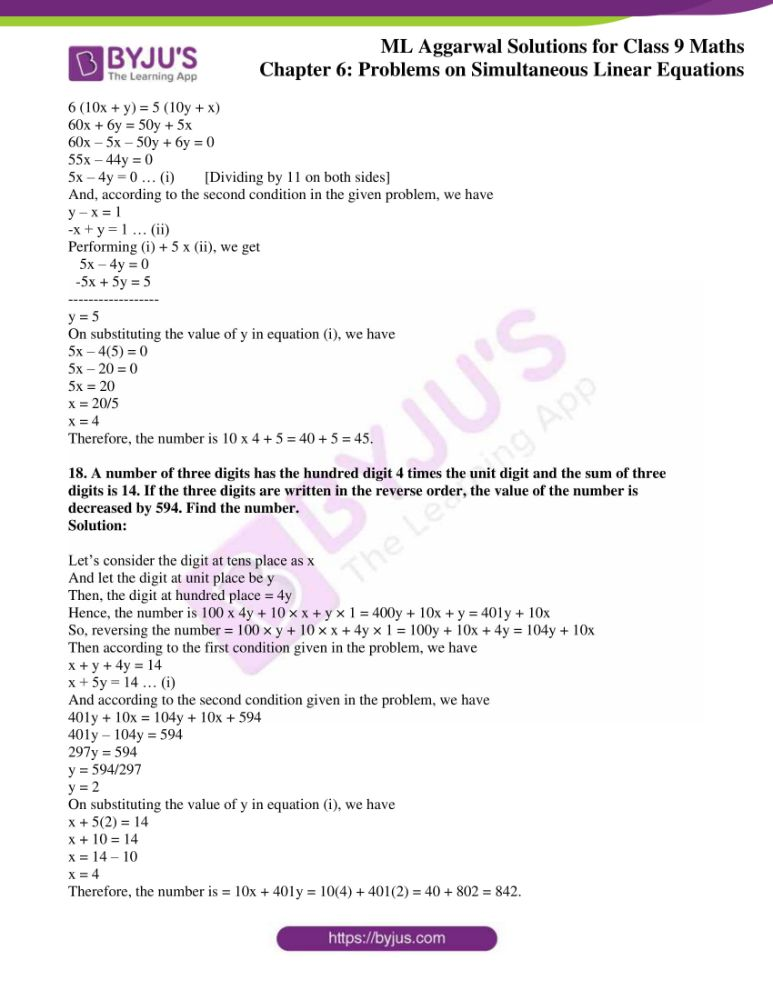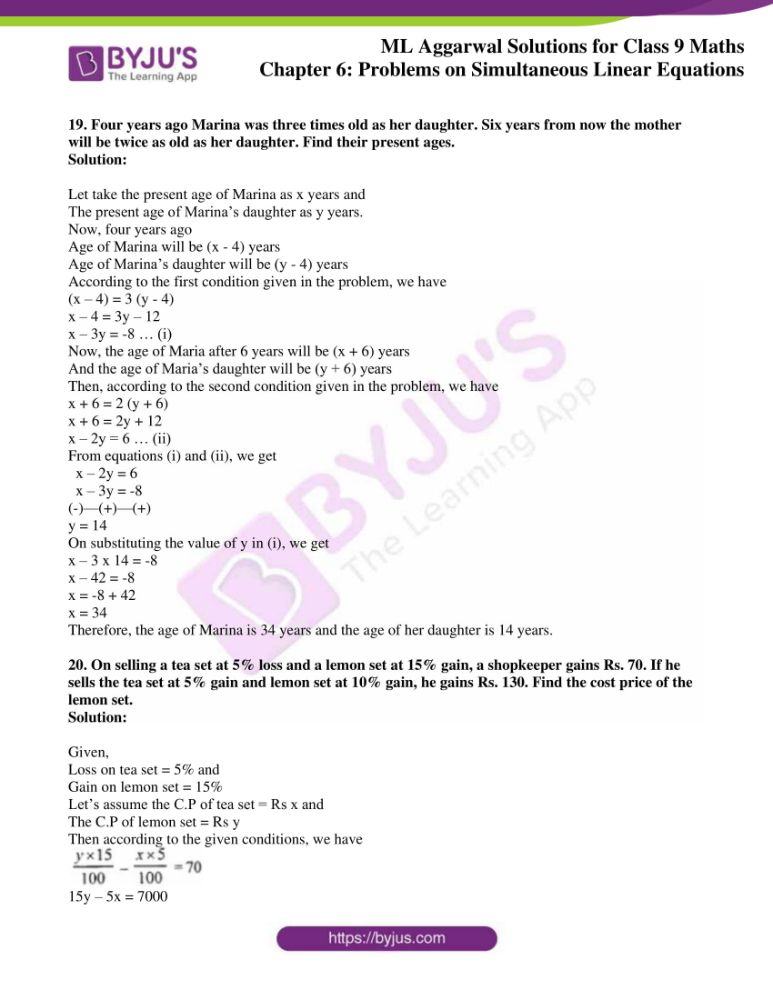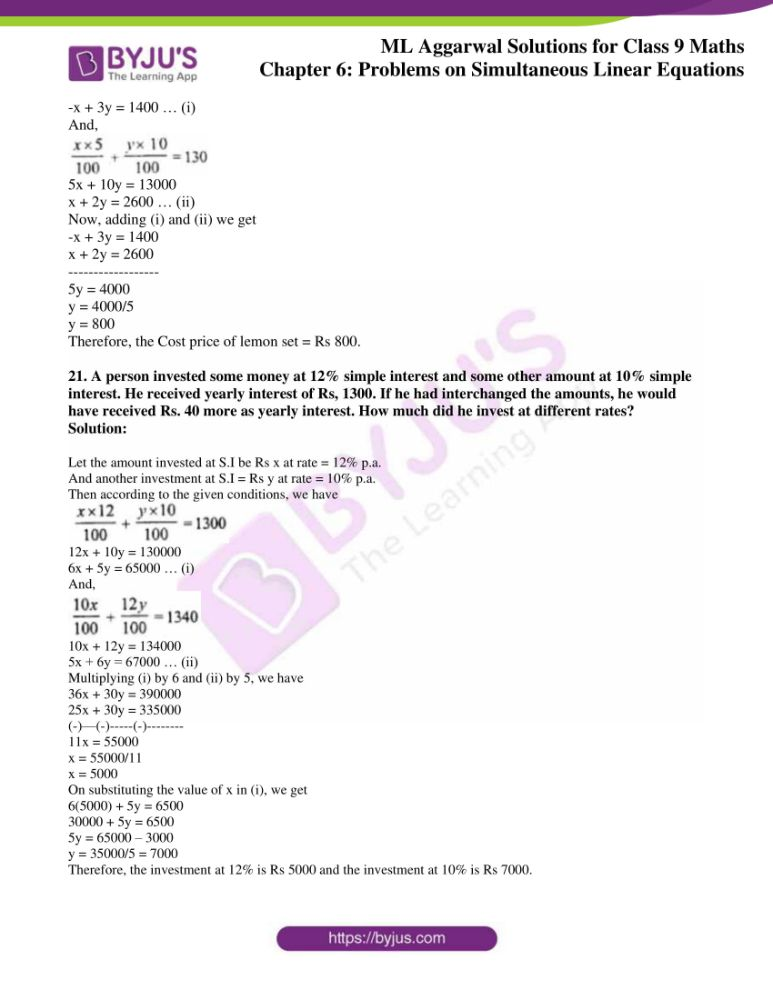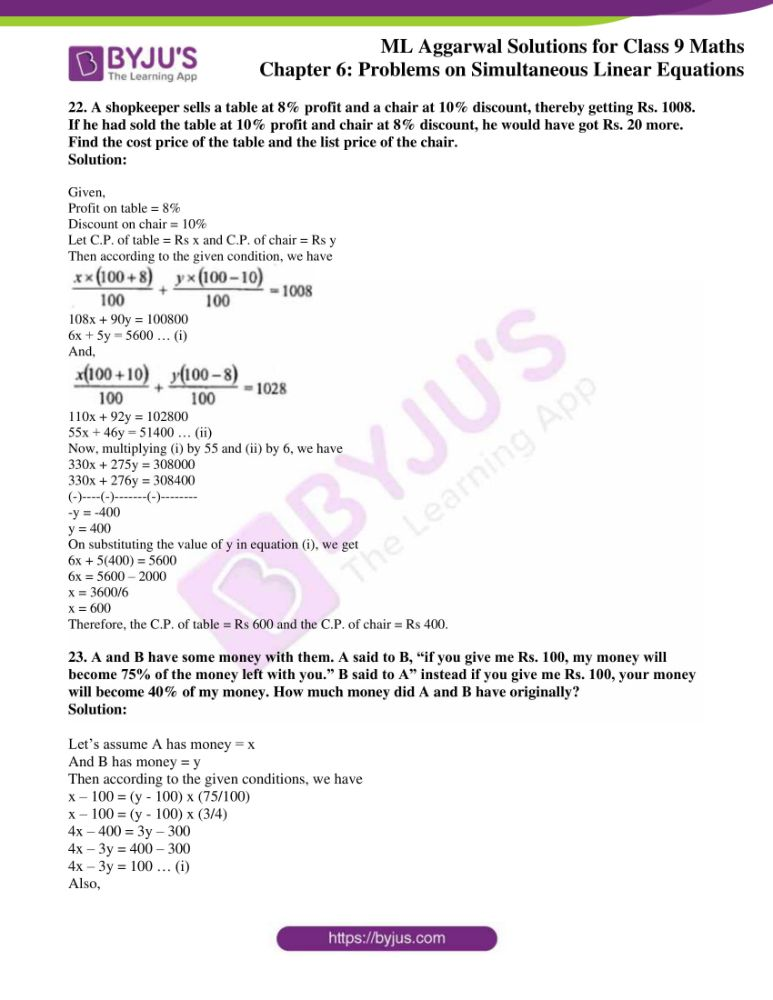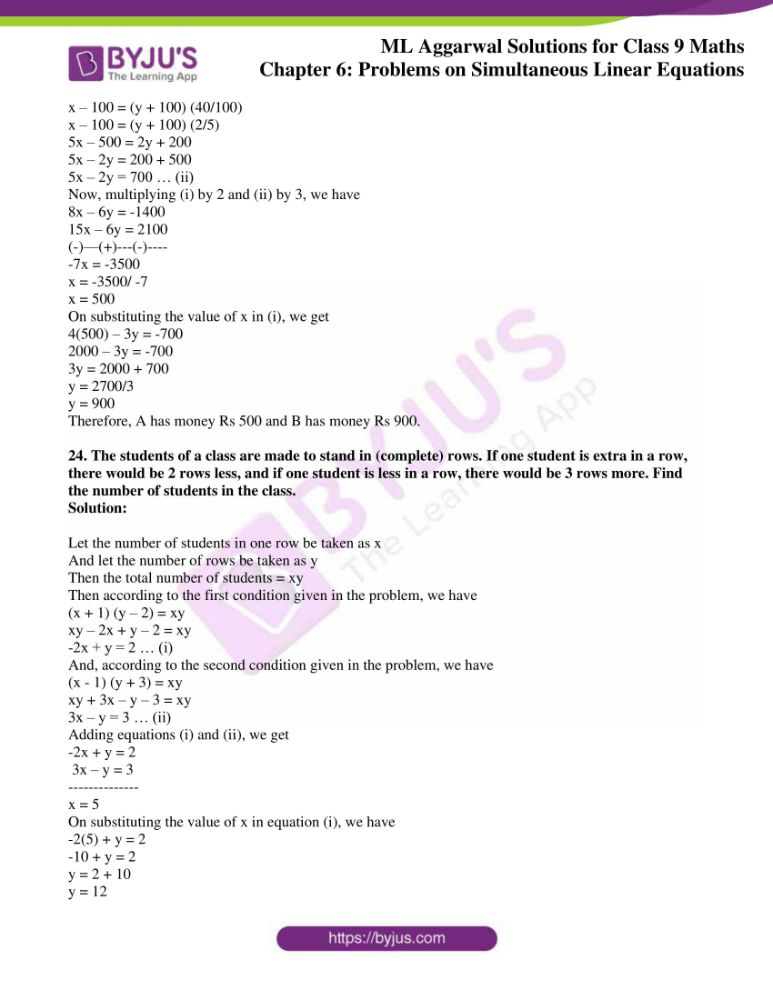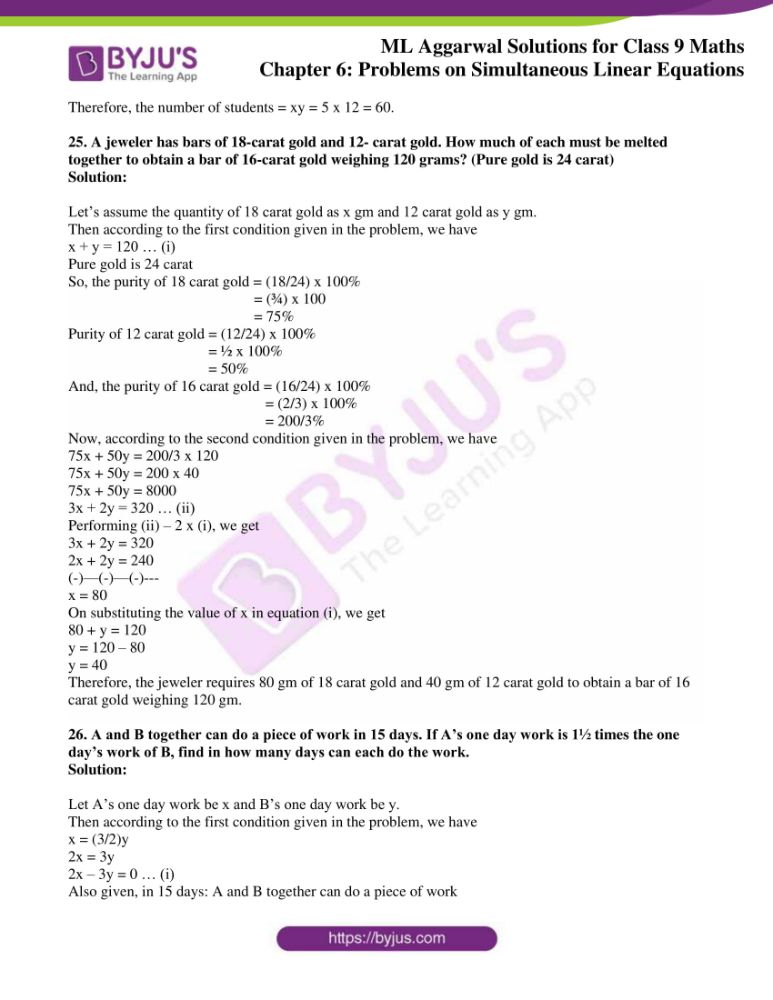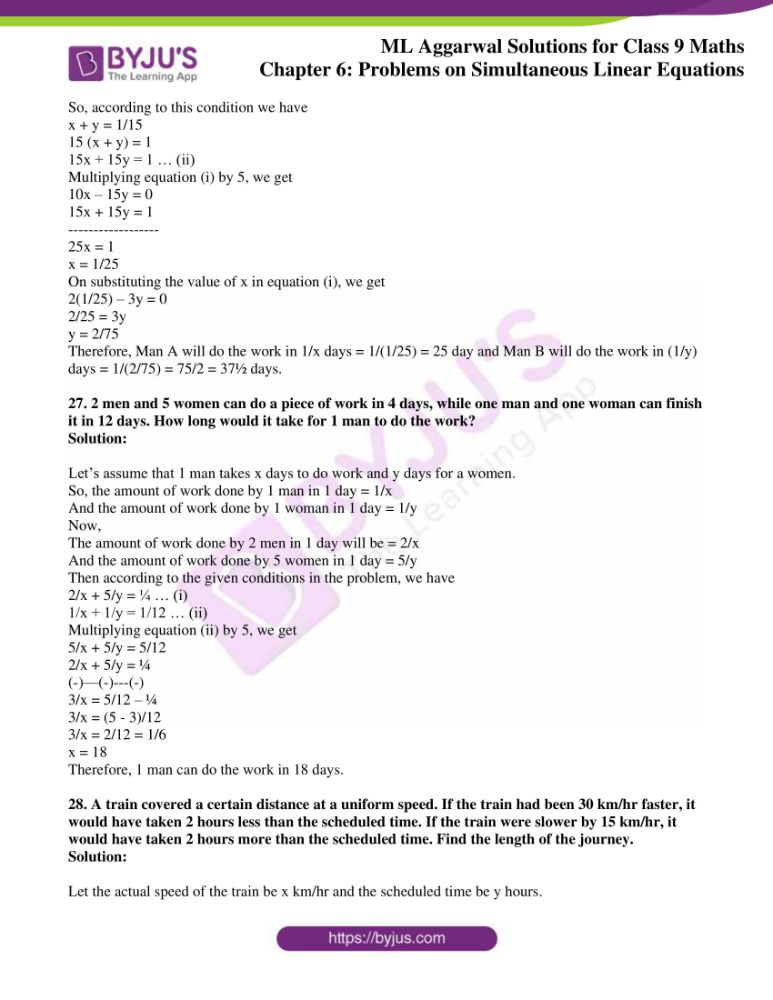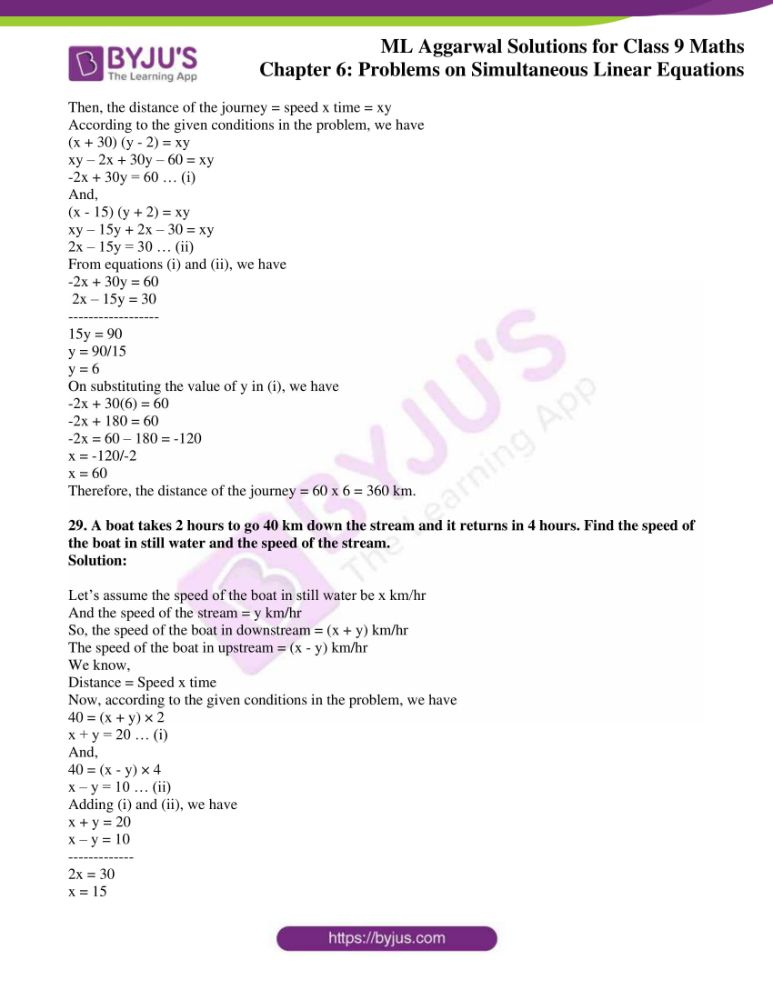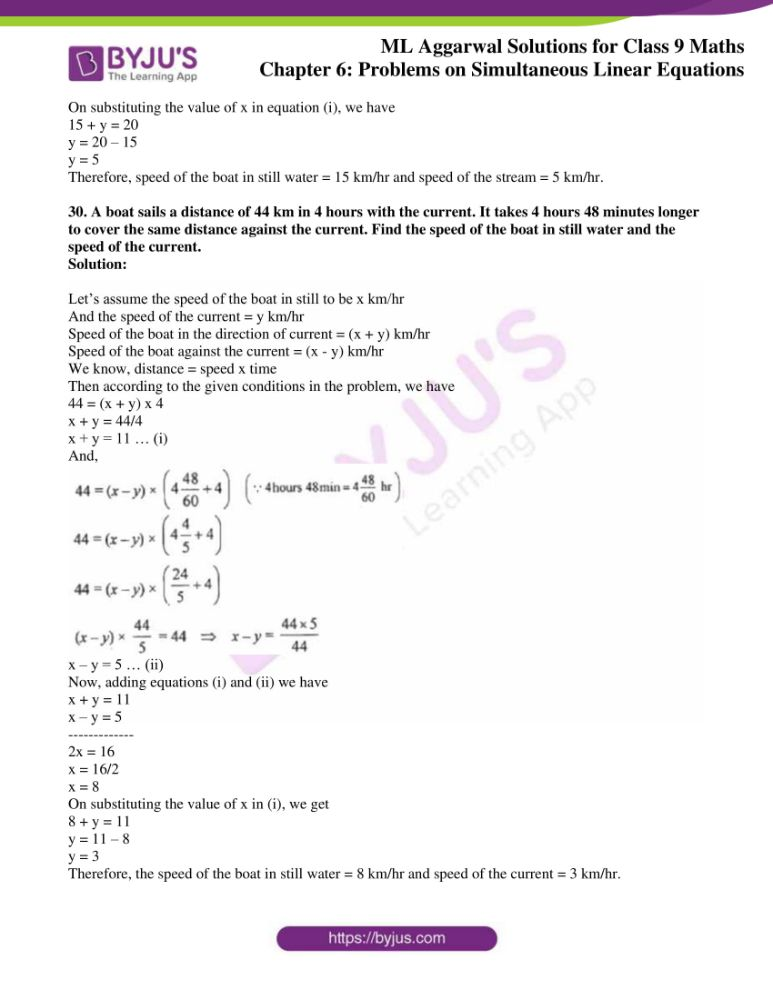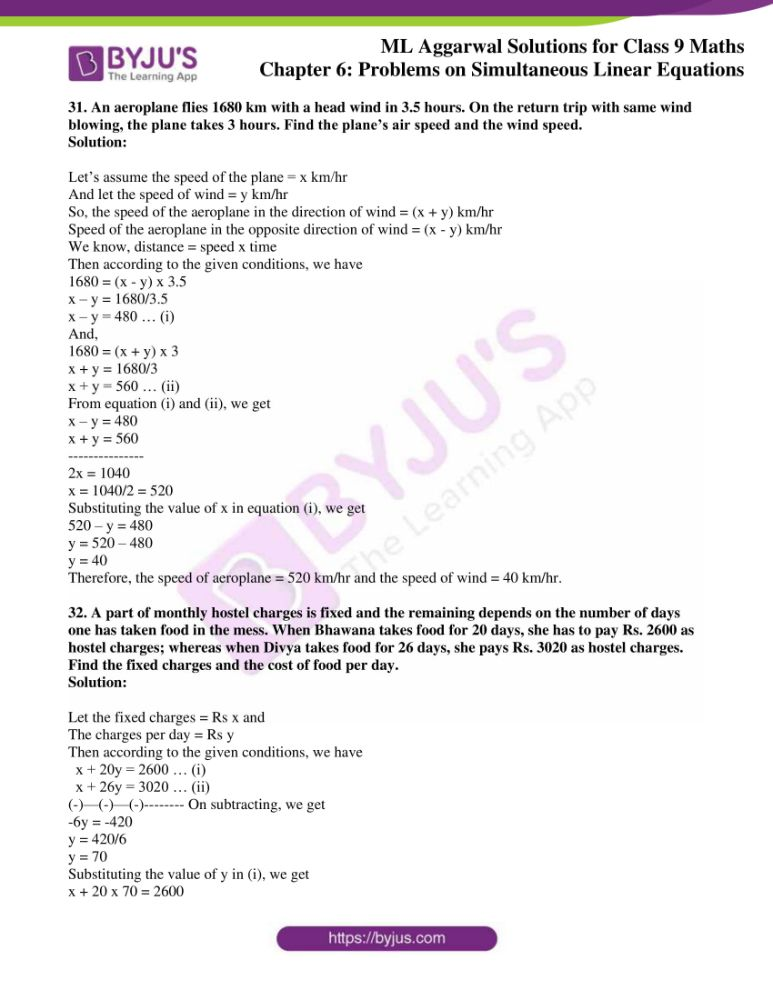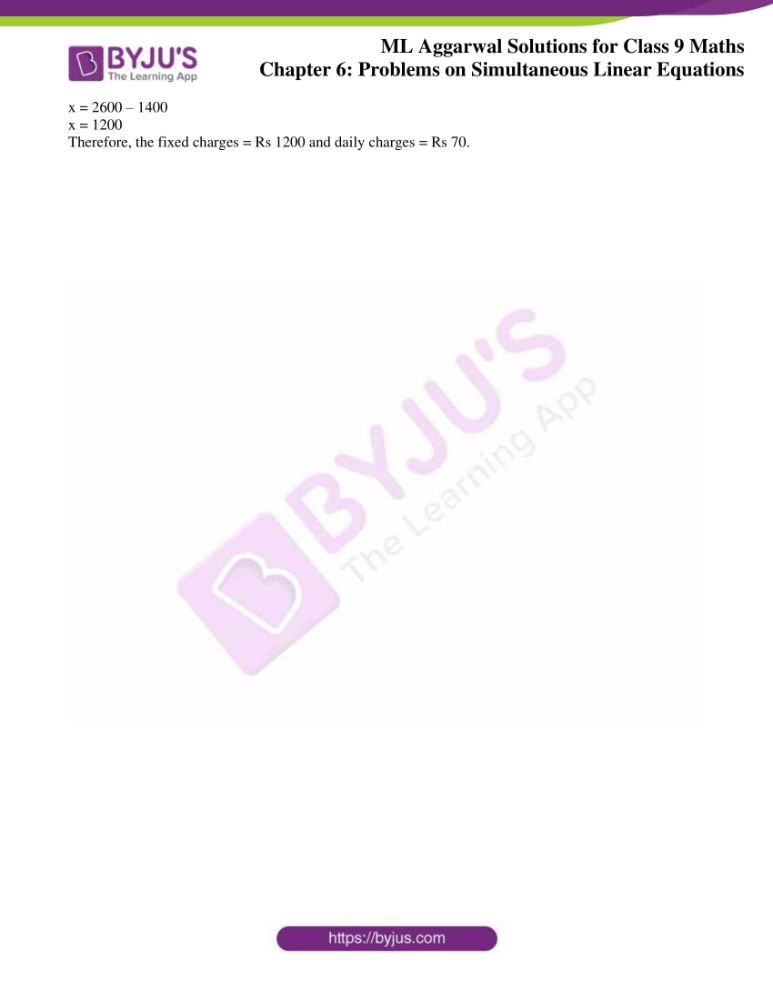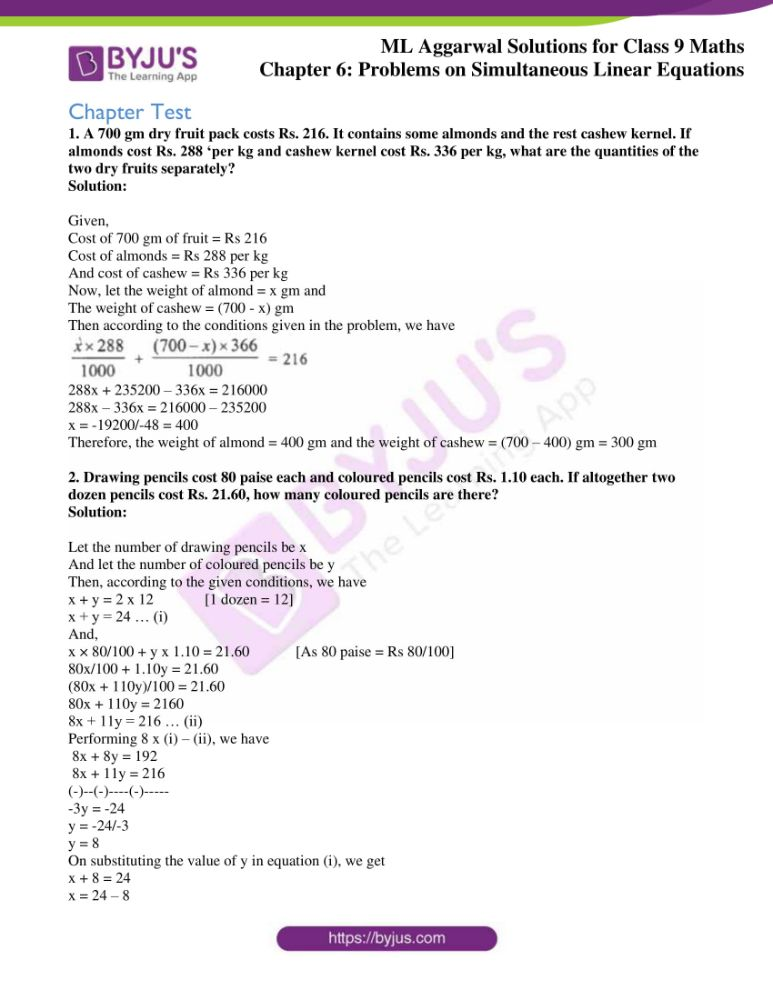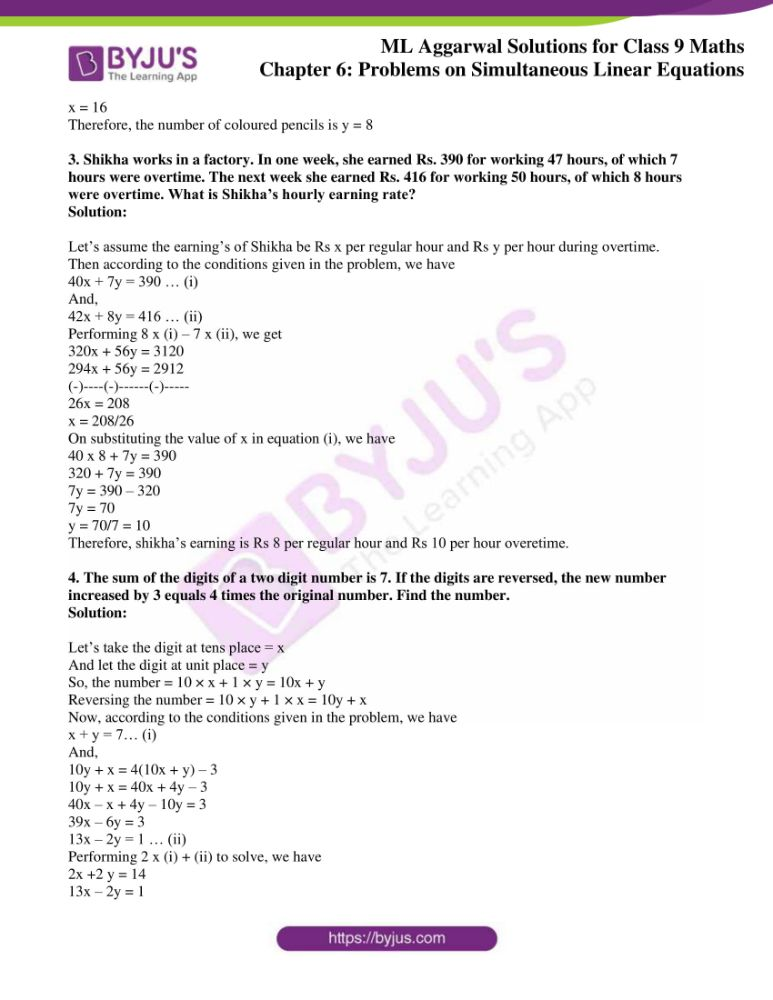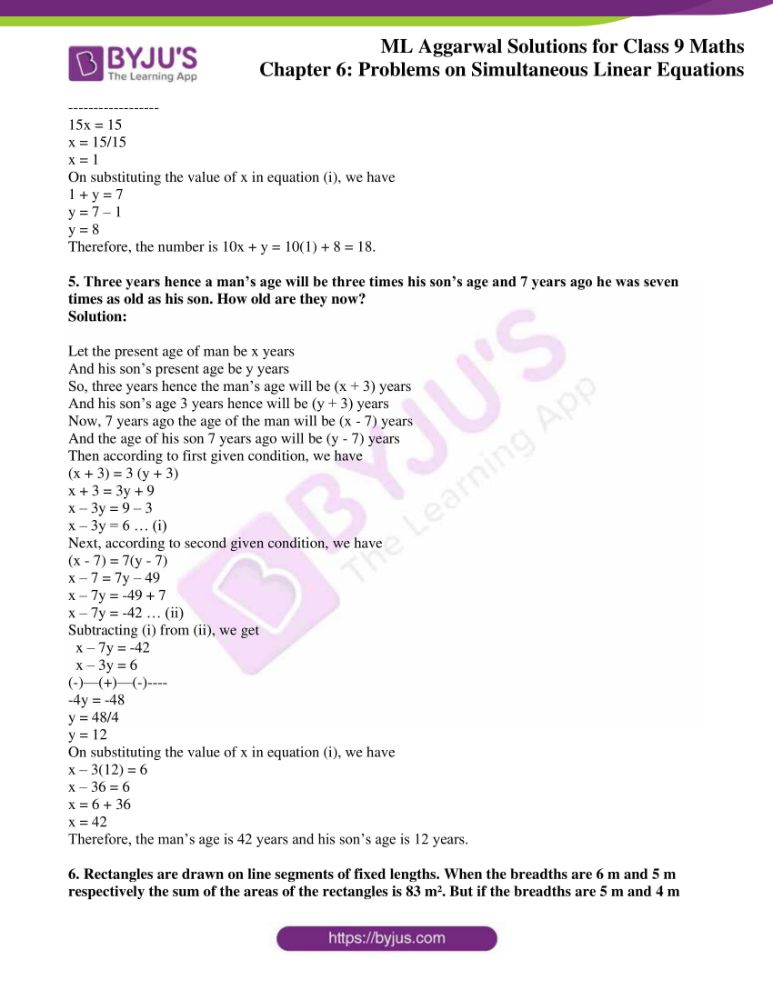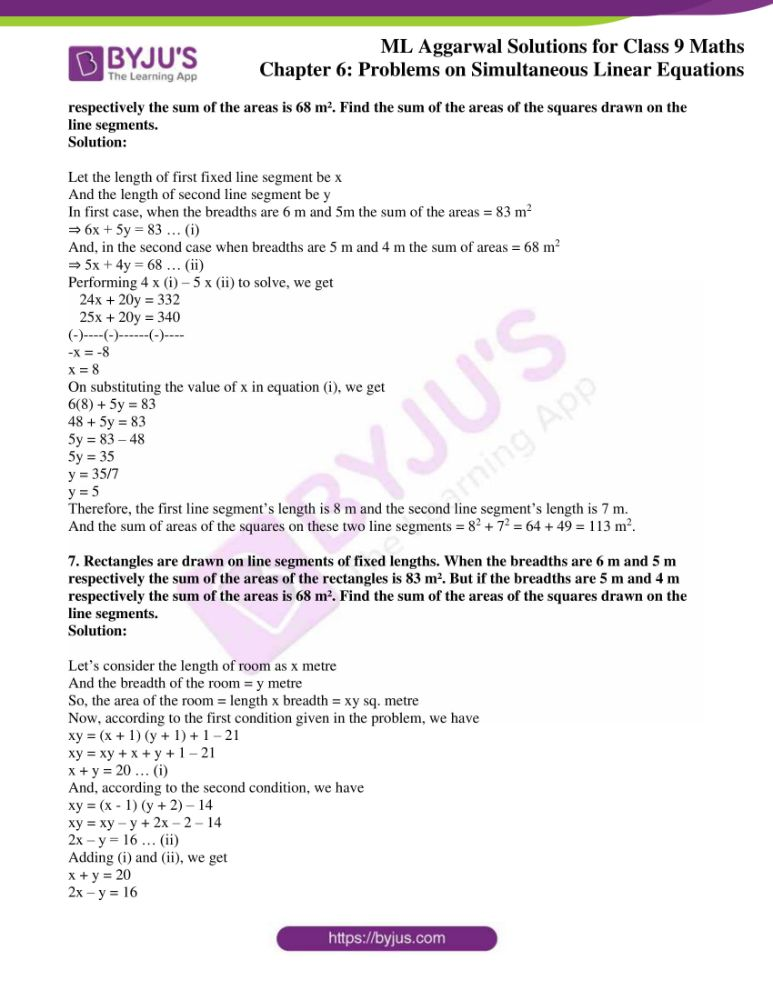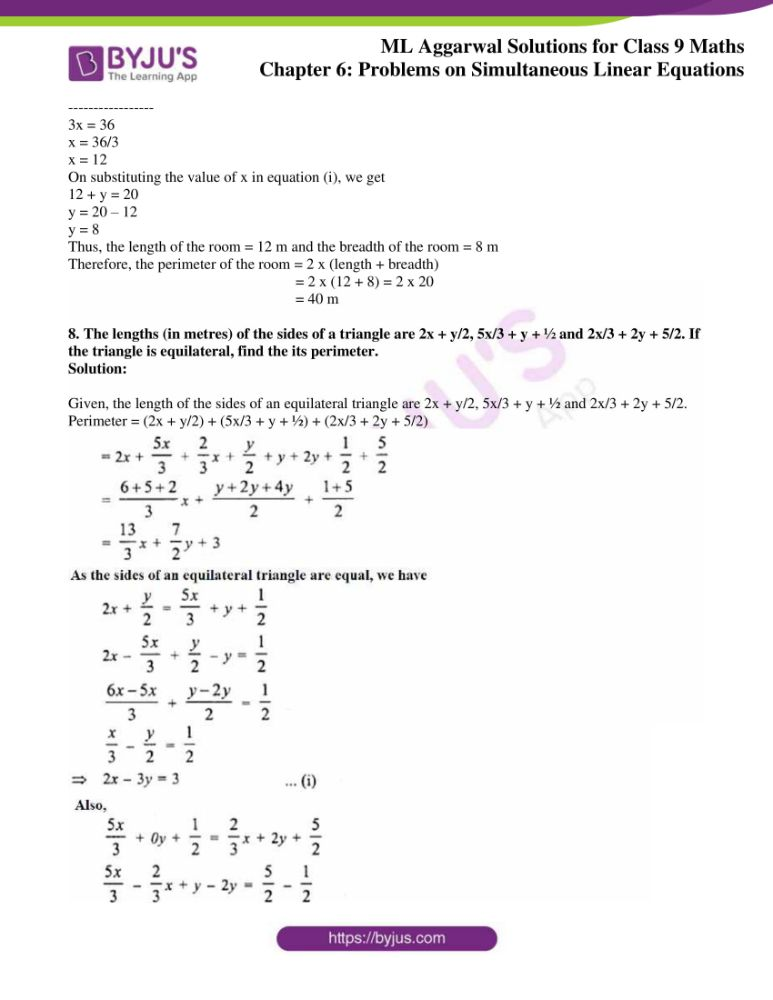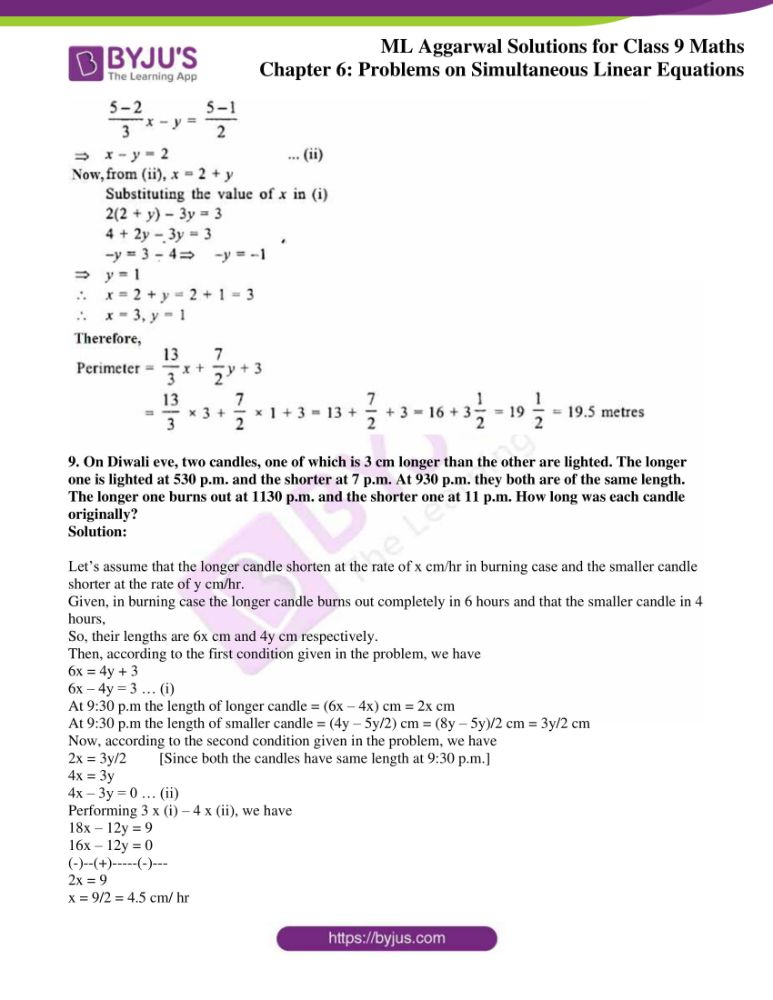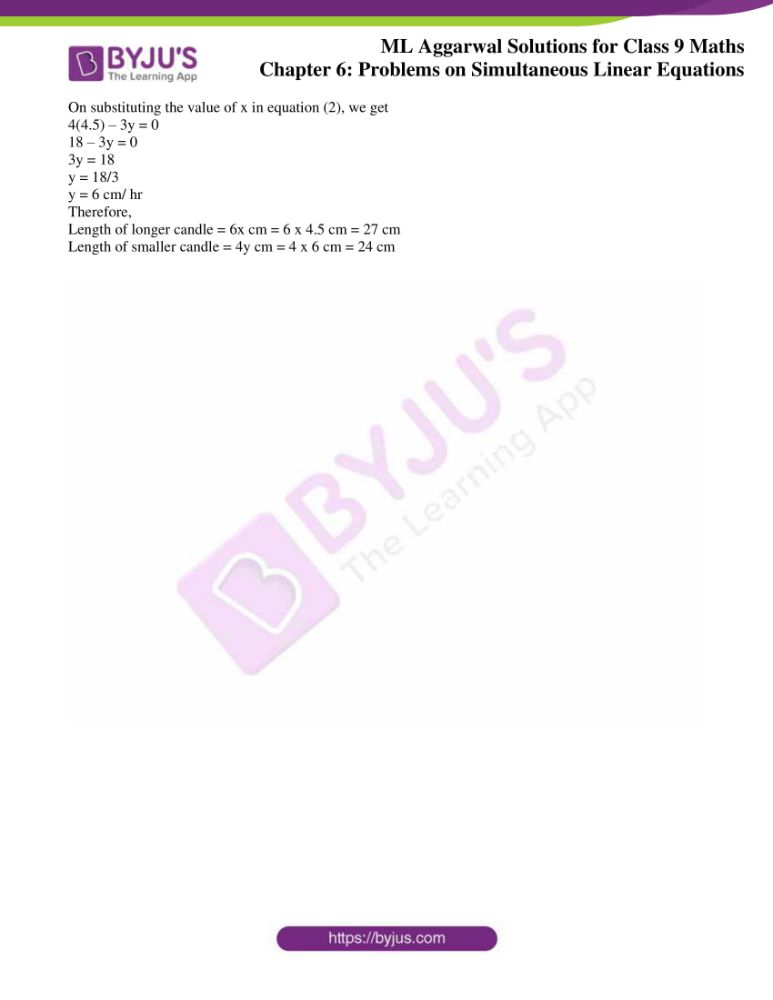## Access ML Aggarwal Solutions for Class 9 Maths Chapter 6 Problems on Simultaneous Linear Equations

Exercise 6

1. The sum of two numbers is 50 and their difference is 16. Find the numbers.

Solution:

Let’s assume the two numbers to be x and y

Then according to the given conditions, we have

x + y = 50 … (i) and

x – y = 16 … (ii)

Now, adding (i) and (ii) we get

2x = 66

x = 33

On substituting the value of x in (i), we get

33 + y = 50

y = 50 – 33

y = 17

Therefore, the two numbers are 33 and 17.

2. The sum of two numbers is 2. If their difference is 20, find the numbers.

Solution:

Let’s assume the two numbers to be x and y

Then according to the given conditions, we have

x + y = 2 … (i) and

x – y = 20 … (ii)

Now, adding (i) and (ii) we get

2x = 22

x = 11

On substituting the value of x in (i), we get

11 + y = 2

y = 2 – 11

y = -9

Therefore, the two numbers are 11 and -9.

3. The sum of two numbers is 43. If the larger is doubled and the smaller is tripled, the difference is 36. Find the two numbers.

Solution:

Let’s assume the two numbers to be x and y such that x > y

Then according to the given conditions, we have

x + y = 43 … (i) and

2x – 3y = 36 … (ii)

Now, multiplying (i) by 3 and adding with (ii) we get

3x + 3y = 129

2x – 3y = 36

—————–

5x = 165

x = 165/5 = 33

On substituting the value of x in (i), we get

33 + y = 43

y = 43 – 33

y = 10

Therefore, the two numbers are 33 and 10.

4. The cost of 5 kg of sugar and 7 kg of rice is Rs. 153, and the cost of 7 kg of sugar and 5 kg of rice is Rs. 147. Find the cost of 6 kg of sugar and 10 kg of rice.

Solution:

Let’s assume the cost of 1 kg of sugar = Rs x

And, let the cost of 1 kg of rice = Rs y

Then according to the given conditions, we have

5x + 7y = 153 … (i) and

7x + 5y = 147 … (ii)

Multiplying (i) by 7 and (ii) by 5, we have

35x + 49y = 1071 … (iii)

35x + 25y = 735 … (iv)

(-)—(-)——(-)————- Subtracting (iv) from (iii), we get

24y = 336

y = 336/24

y = 14

On substituting the value of y in (i), we get

5x + 7(14) = 153

5x + 98 = 153

5x = 153 – 98

5x = 55

x = 55/5 = 11

So, the cost of 1 kg of sugar is Rs 11 and the cost of 1 kg of rice is Rs 14.

Now,

Cost of 6 kg of sugar = Rs 11 x 6 = Rs 66

Cost of 10 kg of rice = Rs 14 x 10 = Rs 140

Thus, the cost of 6 kg of sugar and 10 kg of rice = Rs 66 + Rs 140 = Rs 206.

5. The class IX students of a certain public school wanted to give a farewell party to the outgoing students of class X. They decided to purchase two kinds of sweets, one costing Rs. 70 per kg and the other costing Rs. 84 per kg. They estimated that 36 kg of sweets were needed. If the total money spent on sweets was Rs. 2800, find how much sweets of each kind they purchased.

Solution:

Let the quantity of sweet costing Rs 70 be x

And, the quantity of sweet costing Rs 84 be y

Given, total quantity of sweets purchased is 34 kg

x + y = 34 … (i)

Also given, the total money spent is Rs 2800

70x + 84y = 2800 … (ii)

Multiplying (i) by 70 and subtracting with (ii), we get

70x + 70y = 2520

70x + 84y = 2800

(-)—(-)—–(-)——

-14y = -280

y = -280/-14

y = 20

On substituting the value of y in equation (i), we get

x + 20 = 36

x = 36 – 20

x = 16

Therefore, the quantities of sweets purchased are 16 kg which costs Rs 70 per kg and 20 kg which costs Rs 84 per kg.

6. If from twice the greater of two numbers 16 is subtracted, the result is half the other number. If from half the greater number 1 is subtracted, the result is still half the other number. What are the numbers.

Solution:

Let’s consider the greater number to be x and the smaller number to be y.

Then, according to the given conditions we have

2x – 16 = y/2 ⇒ 4x – 32 = y ⇒ 4x – y = 32 … (i)

And,

x/2 – 1 = y/2 ⇒ x – 2 = y ⇒ x – y = 2 … (ii)

Now, subtracting (ii) from (i) we get

4x – y = 32

x – y = 2

(-)–(+)—(-)—

3x = 30

x = 10

On substituting the value of x in (i), we get

2(10) – 16 = y/2

20 – 16 = y/2

4 = y/2

y = 8

Therefore, the two numbers are 10 and 8.

7. There are 38 coins in a collection of 20 paise coins and 25 paise coins. If the total value of the collection is Rs. 8.50, how many of each are there?

Solution:

Let the number of 20 paise coins be x

And let the number of 25 paise coins be y.

Then, according to the given conditions we have

x + y = 38 … (i) and

20x + 25y = 850 … (ii) [Since, 8.50 Rs = 850 paise]

Performing (ii) – 20 x (i), we get

20x + 25y = 850

20x + 20y = 760

(-)–(-)—–(-)—–

5y = 90

y = 90/5

y = 18

Substituting the value of y in (i), we have

x + 18 = 38

x = 38 – 18

x = 20

Therefore, the number of 20 paise coins are 20 and the number of 25 paise coins are 18.

8. A man has certain notes of denominations Rs. 20 and Rs. 5 which amount to Rs. 380. If the number of notes of each kind is interchanged, they amount to Rs. 60 less as before. Find the number of notes of each denomination.

Solution:

Let’s consider the number of 20 rupee notes to be x

And the number of 5 rupee notes be y

Then, according to the given conditions we have

20x + 5y = 380 … (i) and

5x + 20y = 380 – 60

⇒ 5x + 20 y = 320 … (ii)

Now, multiplying (i) by 4 and subtracting with (ii) we get

80x + 20y = 1520

5x + 20 y = 320

(-)–(-)—-(-)——-

75x = 1200

x = 1200/75

x = 16

On substituting the value of x in (i), we get

20(16) + 5y = 380

320 + 5y = 380

5y = 380 – 320 = 60

y = 60/5

y = 12

Therefore, number of 20 rupee notes = 16 and number of 5 rupee notes = 12

9. The ratio of two numbers is 2/3. If 2 is subtracted from the first and 8 from the second, the ratio becomes the reciprocal of the original ratio. Find the numbers.

Solution:

Let’s assume the two numbers to be x and y.

Given that the ratio of the numbers = 2/3

Then,

x/y = 2/3

3x = 2y

⇒ 3x – 2y = 0 … (i)

Also given, if 2 is subtracted from the first and 8 from the second, the ratio becomes the reciprocal of the original ratio

(x – 2)/(y – 8) = 3/2

2 (x – 2) = 3 (y – 8)

2x – 4 = 3y – 24

⇒ 2x – 3y = -20 … (ii)

Now, performing 3 x (i) – 2 x (ii) we get

9x – 6y = 0

4x – 6y = -40

(-)–(+)—-(+)—

5x = 40

x = 40/5

x = 8

On substituting the value of x in (i), we get

3(8) – 2y = 0

24 = 2y

y = 24/2 = 12

Therefore, the numbers are 8 and 12.

10. If 1 is added to the numerator of a fraction, it becomes 1/5; if 1 is taken from the denominator, it becomes 1/7, find the fraction.

Solution:

Let the fraction be x/y

Then according to the given conditions, we have

(x + 1)/y = 1/5

5 (x + 1) = y

5x + 5 = y

⇒ 5x – y = -5 … (i)

And,

x/(y – 1) = 1/7

7x = y – 1

7x – y = -1 … (ii)

Now, subtracting (i) from (ii) we get

7x – y = -1

5x – y = -5

(-)–(+)-(+)—

2x = 4

x = 2

On substituting the value of x in (i), we get

5(2) – y = -5

10 – y = -5

y = 10 + 5

y = 15

Therefore, the fraction is 2/15.

11. If the numerator of a certain fraction is increased by 2 and the denominator by 1, the fraction becomes equal to 5/8 and if the numerator and denominator are each diminished by 1, the fraction becomes equal to ½, find the fraction.

Solution:

Let the fraction be x/y

Then according to the given conditions, we have

(x + 2)/ (y + 1) = 5/8

8 (x + 2) = 5 (y + 1)

8x + 16 = 5y + 5

8x – 5y = 5 – 16

8x – 5y = -11 … (i)

And,

(x – 1)/ (y – 1) = ½

2 (x – 1) = (y – 1)

2x – 2 = y – 1

2x – y = 1 … (ii)

Now, performing (i) – 4 x (ii) we get

8x – 5y = -11

8x – 4y = 4

(-)—(+)—(-)

-y = -15

y = 15

On substituting the value of y in (ii), we get

2x – 15 = 1

2x = 1 + 15

x = 16/2 = 8

Therefore, the fraction is 8/15.

12. Find the fraction which becomes ½ when the denominator is increased by 4 and is equal to 1/8, when the numerator is diminished by 5.

Solution:

Let the fraction be x/y.

Then according to the given conditions, we have

x/ (y + 4) = ½

2x = y + 4

2x – y = 4 … (i)

And,

(x – 5)/ y = 1/8

8 (x – 5) = y

8x – 40 = y

8x – y = 40 … (ii)

Now, subtracting (i) from (ii) we get

8x – y = 40

2x – y = 4

(-)–(+)—(-)—

6x = 36

x = 36/6

x = 6

On substituting the value of x in (i), we get

2(6) – y = 4

12 – y = 4

y = 12 – 4 = 8

Therefore, the fraction is 6/8.

13. In a two-digit number the sum of the digits is 7. If the number with the order of the digits reversed is 28 greater than twice the unit’s digit of the original number, find the number.

Solution:

Let’s consider the digit at tens place as x

And let the digit at unit place be y

Then according to the first condition, we have

x + y = 7 … (i)

Also, the number = 10 × x + y × 1 = 10y + x

Now, according to the second condition we have

10y + x = 2y + 28

x + 8y = 28 … (ii)

Subtracting (i) from (ii), we get

x + 8y = 28

x + y = 7

(-)—(-)—(-)

7y = 21

y = 21/7

y = 3

Substituting the value of y in (i), we get

x + 3 = 7

x = 7 – 3

x = 4

Therefore, the number is 10 x 4 + 3 = 43.

14. A number of two digits exceeds four times the sum of its digits by 6 and it is increased by 9 on reversing the digits. Find the number.

Solution:

Let’s consider the digit at tens place as x

And let the digit at unit place be y

The number is 10 × x + y × 1 = 10x + y

So, reversing the number = 10 × y + x × 1 = 10y + y

Then according to the first condition, we have

10x + y = 4 (x + y) + 6

10x – 4x + y – 4y = 6

6x – 3y = 6

2x – y = 2 … (i)

And according to the second condition, we have

10x + y + 9 = 10y + x

10x – x + y – 10y = -9

9x – 9y = -9

x – y = -1 … (ii)

Now, subtracting (ii) from (i) we have

2x – y = 2

x – y = -1

(-)–(+)–(+)—

x = 3

Substituting the value of x in (i), we get

2(3) – y = 2

6 – y = 2

y = 6 – 2

y = 4

Therefore, the number is 10 x 3 + 4 = 30 + 4 = 34.

15. When a two-digit number is divided by the sum of its digits the quotient is 8. If the ten’s digit is diminished by three times the unit’s digit the remainder is 1. What is the number?

Solution:

Let’s consider the digit at tens place as x

And let the digit at unit place be y

The number is 10 × x + y × 1 = 10x + y

Then according to the first condition, we have

(10x + y)/ (x + y) = 8

10x + y = 8 (x + y)

10x + y = 8x + 8y

10x – 8x + y – 8y = 0

2x – 7y = 0 … (i)

And according to the second condition, we have

x – 3y = 1 … (ii)

Performing (i) – 2 x (ii), we get

2x – 7y = 0

2x – 6y = 2

(-)—(+)—(-)

-y = -2

y = 2

Substituting the value of y in (i), we get

2x – 7(2) = 0

2x = 14

x = 14/2

x = 7

Therefore, the number is = 10 x 7 + 2 = 70 + 2 = 72.

16. The result of dividing a number of two digits by the number with digits reversed is 1¾. If the sum of digits is 12, find the number.

Solution:

Let’s consider the digit at tens place as x

And let the digit at unit place be y

The number is 10 × x + y × 1 = 10x + y

So, reversing the number = 10 × y + x × 1 = 10y + y

Then according to the first condition given in the problem, we have

(10x + y)/ (10y + x) = 1¾

(10x + y)/ (10y + x) = 7/4

4 (10x + y) = 7 (10y + x)

40x + 4y = 70y + 7x

40x – 7x – 70y + 4y = 0

33x – 66y = 0

x – 2y = 0 … (i)

And according to the second condition of the given problem, we have

x + y = 12 … (ii)

From equation (i) and (ii), we have

x + y = 12

x – 2y = 0

(-)—(+)—(-)

3y = 12

y = 12/3

y = 4

On substituting the value of y in (i), we have

x – 2(4) = 0

x = 8

Therefore, the number is 10 x 8 + 4 = 84.

17. The result of dividing a number of two digits by the number with the digits reversed is 5/6. If the difference of digits is 1, find the number.

Solution:

Let’s consider the digit at tens place as x

And let the digit at unit place be y

The number is 10 × x + y × 1 = 10x + y

So, reversing the number = 10 × y + x × 1 = 10y + y

Then according to the first condition given in the problem, we have

(10x + y)/ (10y + x) = 5/6

6 (10x + y) = 5 (10y + x)

60x + 6y = 50y + 5x

60x – 5x – 50y + 6y = 0

55x – 44y = 0

5x – 4y = 0 … (i) [Dividing by 11 on both sides]

And, according to the second condition in the given problem, we have

y – x = 1

-x + y = 1 … (ii)

Performing (i) + 5 x (ii), we get

5x – 4y = 0

-5x + 5y = 5

——————

y = 5

On substituting the value of y in equation (i), we have

5x – 4(5) = 0

5x – 20 = 0

5x = 20

x = 20/5

x = 4

Therefore, the number is 10 x 4 + 5 = 40 + 5 = 45.

18. A number of three digits has the hundred digit 4 times the unit digit and the sum of three digits is 14. If the three digits are written in the reverse order, the value of the number is decreased by 594. Find the number.

Solution:

Let’s consider the digit at tens place as x

And let the digit at unit place be y

Then, the digit at hundred place = 4y

Hence, the number is 100 x 4y + 10 × x + y × 1 = 400y + 10x + y = 401y + 10x

So, reversing the number = 100 × y + 10 × x + 4y × 1 = 100y + 10x + 4y = 104y + 10x

Then according to the first condition given in the problem, we have

x + y + 4y = 14

x + 5y = 14 … (i)

And according to the second condition given in the problem, we have

401y + 10x = 104y + 10x + 594

401y – 104y = 594

297y = 594

y = 594/297

y = 2

On substituting the value of y in equation (i), we have

x + 5(2) = 14

x + 10 = 14

x = 14 – 10

x = 4

Therefore, the number is = 10x + 401y = 10(4) + 401(2) = 40 + 802 = 842.

19. Four years ago Marina was three times old as her daughter. Six years from now the mother will be twice as old as her daughter. Find their present ages.

Solution:

Let take the present age of Marina as x years and

The present age of Marina’s daughter as y years.

Now, four years ago

Age of Marina will be (x – 4) years

Age of Marina’s daughter will be (y – 4) years

According to the first condition given in the problem, we have

(x – 4) = 3 (y – 4)

x – 4 = 3y – 12

x – 3y = -8 … (i)

Now, the age of Maria after 6 years will be (x + 6) years

And the age of Maria’s daughter will be (y + 6) years

Then, according to the second condition given in the problem, we have

x + 6 = 2 (y + 6)

x + 6 = 2y + 12

x – 2y = 6 … (ii)

From equations (i) and (ii), we get

x – 2y = 6

x – 3y = -8

(-)—(+)—(+)

y = 14

On substituting the value of y in (i), we get

x – 3 x 14 = -8

x – 42 = -8

x = -8 + 42

x = 34

Therefore, the age of Marina is 34 years and the age of her daughter is 14 years.

20. On selling a tea set at 5% loss and a lemon set at 15% gain, a shopkeeper gains Rs. 70. If he sells the tea set at 5% gain and lemon set at 10% gain, he gains Rs. 130. Find the cost price of the lemon set.

Solution:

Given,

Loss on tea set = 5% and

Gain on lemon set = 15%

Let’s assume the C.P of tea set = Rs x and

The C.P of lemon set = Rs y

Then according to the given conditions, we have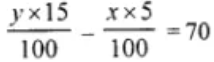15y – 5x = 7000

-x + 3y = 1400 … (i)

And,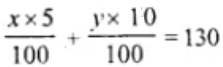5x + 10y = 13000

x + 2y = 2600 … (ii)

Now, adding (i) and (ii) we get

-x + 3y = 1400

x + 2y = 2600

——————

5y = 4000

y = 4000/5

y = 800

Therefore, the Cost price of lemon set = Rs 800.

21. A person invested some money at 12% simple interest and some other amount at 10% simple interest. He received yearly interest of Rs, 1300. If he had interchanged the amounts, he would have received Rs. 40 more as yearly interest. How much did he invest at different rates?

Solution:

Let the amount invested at S.I be Rs x at rate = 12% p.a.

And another investment at S.I = Rs y at rate = 10% p.a.

Then according to the given conditions, we have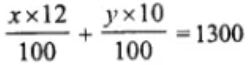12x + 10y = 130000

6x + 5y = 65000 … (i)

And,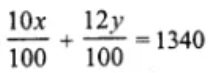10x + 12y = 134000

5x + 6y = 67000 … (ii)

Multiplying (i) by 6 and (ii) by 5, we have

36x + 30y = 390000

25x + 30y = 335000

(-)—(-)—–(-)——–

11x = 55000

x = 55000/11

x = 5000

On substituting the value of x in (i), we get

6(5000) + 5y = 6500

30000 + 5y = 6500

5y = 65000 – 3000

y = 35000/5 = 7000

Therefore, the investment at 12% is Rs 5000 and the investment at 10% is Rs 7000.

22. A shopkeeper sells a table at 8% profit and a chair at 10% discount, thereby getting Rs. 1008. If he had sold the table at 10% profit and chair at 8% discount, he would have got Rs. 20 more. Find the cost price of the table and the list price of the chair.

Solution:

Given,

Profit on table = 8%

Discount on chair = 10%

Let C.P. of table = Rs x and C.P. of chair = Rs y

Then according to the given condition, we have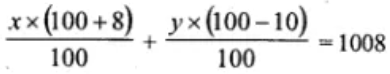108x + 90y = 100800

6x + 5y = 5600 … (i)

And,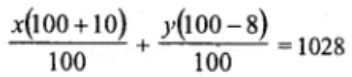110x + 92y = 102800

55x + 46y = 51400 … (ii)

Now, multiplying (i) by 55 and (ii) by 6, we have

330x + 275y = 308000

330x + 276y = 308400

(-)—-(-)——-(-)——–

-y = -400

y = 400

On substituting the value of y in equation (i), we get

6x + 5(400) = 5600

6x = 5600 – 2000

x = 3600/6

x = 600

Therefore, the C.P. of table = Rs 600 and the C.P. of chair = Rs 400.

23. A and B have some money with them. A said to B, “if you give me Rs. 100, my money will become 75% of the money left with you.” B said to A” instead if you give me Rs. 100, your money will become 40% of my money. How much money did A and B have originally?

Solution:

Let’s assume A has money = x

And B has money = y

Then according to the given conditions, we have

x – 100 = (y – 100) x (75/100)

x – 100 = (y – 100) x (3/4)

4x – 400 = 3y – 300

4x – 3y = 400 – 300

4x – 3y = 100 … (i)

Also,

x – 100 = (y + 100) (40/100)

x – 100 = (y + 100) (2/5)

5x – 500 = 2y + 200

5x – 2y = 200 + 500

5x – 2y = 700 … (ii)

Now, multiplying (i) by 2 and (ii) by 3, we have

8x – 6y = -1400

15x – 6y = 2100

(-)—(+)—(-)—-

-7x = -3500

x = -3500/ -7

x = 500

On substituting the value of x in (i), we get

4(500) – 3y = -700

2000 – 3y = -700

3y = 2000 + 700

y = 2700/3

y = 900

Therefore, A has money Rs 500 and B has money Rs 900.

24. The students of a class are made to stand in (complete) rows. If one student is extra in a row, there would be 2 rows less, and if one student is less in a row, there would be 3 rows more. Find the number of students in the class.

Solution:

Let the number of students in one row be taken as x

And let the number of rows be taken as y

Then the total number of students = xy

Then according to the first condition given in the problem, we have

(x + 1) (y – 2) = xy

xy – 2x + y – 2 = xy

-2x + y = 2 … (i)

And, according to the second condition given in the problem, we have

(x – 1) (y + 3) = xy

xy + 3x – y – 3 = xy

3x – y = 3 … (ii)

Adding equations (i) and (ii), we get

-2x + y = 2

3x – y = 3

————–

x = 5

On substituting the value of x in equation (i), we have

-2(5) + y = 2

-10 + y = 2

y = 2 + 10

y = 12

Therefore, the number of students = xy = 5 x 12 = 60.

25. A jeweler has bars of 18-carat gold and 12- carat gold. How much of each must be melted together to obtain a bar of 16-carat gold weighing 120 grams? (Pure gold is 24 carat)

Solution:

Let’s assume the quantity of 18 carat gold as x gm and 12 carat gold as y gm.

Then according to the first condition given in the problem, we have

x + y = 120 … (i)

Pure gold is 24 carat

So, the purity of 18 carat gold = (18/24) x 100%

= (¾) x 100

= 75%

Purity of 12 carat gold = (12/24) x 100%

= ½ x 100%

= 50%

And, the purity of 16 carat gold = (16/24) x 100%

= (2/3) x 100%

= 200/3%

Now, according to the second condition given in the problem, we have

75x + 50y = 200/3 x 120

75x + 50y = 200 x 40

75x + 50y = 8000

3x + 2y = 320 … (ii)

Performing (ii) – 2 x (i), we get

3x + 2y = 320

2x + 2y = 240

(-)—(-)—(-)—

x = 80

On substituting the value of x in equation (i), we get

80 + y = 120

y = 120 – 80

y = 40

Therefore, the jeweler requires 80 gm of 18 carat gold and 40 gm of 12 carat gold to obtain a bar of 16 carat gold weighing 120 gm.

26. A and B together can do a piece of work in 15 days. If A’s one day work is 1½ times the one day’s work of B, find in how many days can each do the work.

Solution:

Let A’s one day work be x and B’s one day work be y.

Then according to the first condition given in the problem, we have

x = (3/2)y

2x = 3y

2x – 3y = 0 … (i)

Also given, in 15 days: A and B together can do a piece of work

So, according to this condition we have

x + y = 1/15

15 (x + y) = 1

15x + 15y = 1 … (ii)

Multiplying equation (i) by 5, we get

10x – 15y = 0

15x + 15y = 1

——————

25x = 1

x = 1/25

On substituting the value of x in equation (i), we get

2(1/25) – 3y = 0

2/25 = 3y

y = 2/75

Therefore, Man A will do the work in 1/x days = 1/(1/25) = 25 day and Man B will do the work in (1/y) days = 1/(2/75) = 75/2 = 37½ days.

27. 2 men and 5 women can do a piece of work in 4 days, while one man and one woman can finish it in 12 days. How long would it take for 1 man to do the work?

Solution:

Let’s assume that 1 man takes x days to do work and y days for a women.

So, the amount of work done by 1 man in 1 day = 1/x

And the amount of work done by 1 woman in 1 day = 1/y

Now,

The amount of work done by 2 men in 1 day will be = 2/x

And the amount of work done by 5 women in 1 day = 5/y

Then according to the given conditions in the problem, we have

2/x + 5/y = ¼ … (i)

1/x + 1/y = 1/12 … (ii)

Multiplying equation (ii) by 5, we get

5/x + 5/y = 5/12

2/x + 5/y = ¼

(-)—(-)—(-)

3/x = 5/12 – ¼

3/x = (5 – 3)/12

3/x = 2/12 = 1/6

x = 18

Therefore, 1 man can do the work in 18 days.

28. A train covered a certain distance at a uniform speed. If the train had been 30 km/hr faster, it would have taken 2 hours less than the scheduled time. If the train were slower by 15 km/hr, it would have taken 2 hours more than the scheduled time. Find the length of the journey.

Solution:

Let the actual speed of the train be x km/hr and the scheduled time be y hours.

Then, the distance of the journey = speed x time = xy

According to the given conditions in the problem, we have

(x + 30) (y – 2) = xy

xy – 2x + 30y – 60 = xy

-2x + 30y = 60 … (i)

And,

(x – 15) (y + 2) = xy

xy – 15y + 2x – 30 = xy

2x – 15y = 30 … (ii)

From equations (i) and (ii), we have

-2x + 30y = 60

2x – 15y = 30

——————

15y = 90

y = 90/15

y = 6

On substituting the value of y in (i), we have

-2x + 30(6) = 60

-2x + 180 = 60

-2x = 60 – 180 = -120

x = -120/-2

x = 60

Therefore, the distance of the journey = 60 x 6 = 360 km.

29. A boat takes 2 hours to go 40 km down the stream and it returns in 4 hours. Find the speed of the boat in still water and the speed of the stream.

Solution:

Let’s assume the speed of the boat in still water be x km/hr

And the speed of the stream = y km/hr

So, the speed of the boat in downstream = (x + y) km/hr

The speed of the boat in upstream = (x – y) km/hr

We know,

Distance = Speed x time

Now, according to the given conditions in the problem, we have

40 = (x + y) × 2

x + y = 20 … (i)

And,

40 = (x – y) × 4

x – y = 10 … (ii)

Adding (i) and (ii), we have

x + y = 20

x – y = 10

————-

2x = 30

x = 15

On substituting the value of x in equation (i), we have

15 + y = 20

y = 20 – 15

y = 5

Therefore, speed of the boat in still water = 15 km/hr and speed of the stream = 5 km/hr.

30. A boat sails a distance of 44 km in 4 hours with the current. It takes 4 hours 48 minutes longer to cover the same distance against the current. Find the speed of the boat in still water and the speed of the current.

Solution:

Let’s assume the speed of the boat in still to be x km/hr

And the speed of the current = y km/hr

Speed of the boat in the direction of current = (x + y) km/hr

Speed of the boat against the current = (x – y) km/hr

We know, distance = speed x time

Then according to the given conditions in the problem, we have

44 = (x + y) x 4

x + y = 44/4

x + y = 11 … (i)

And,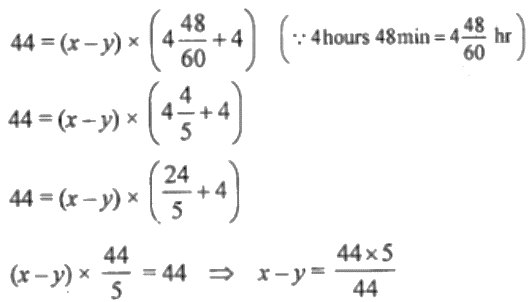x – y = 5 … (ii)

Now, adding equations (i) and (ii) we have

x + y = 11

x – y = 5

————-

2x = 16

x = 16/2

x = 8

On substituting the value of x in (i), we get

8 + y = 11

y = 11 – 8

y = 3

Therefore, the speed of the boat in still water = 8 km/hr and speed of the current = 3 km/hr.

31. An aeroplane flies 1680 km with a head wind in 3.5 hours. On the return trip with same wind blowing, the plane takes 3 hours. Find the plane’s air speed and the wind speed.

Solution:

Let’s assume the speed of the plane = x km/hr

And let the speed of wind = y km/hr

So, the speed of the aeroplane in the direction of wind = (x + y) km/hr

Speed of the aeroplane in the opposite direction of wind = (x – y) km/hr

We know, distance = speed x time

Then according to the given conditions, we have

1680 = (x – y) x 3.5

x – y = 1680/3.5

x – y = 480 … (i)

And,

1680 = (x + y) x 3

x + y = 1680/3

x + y = 560 … (ii)

From equation (i) and (ii), we get

x – y = 480

x + y = 560

—————

2x = 1040

x = 1040/2 = 520

Substituting the value of x in equation (i), we get

520 – y = 480

y = 520 – 480

y = 40

Therefore, the speed of aeroplane = 520 km/hr and the speed of wind = 40 km/hr.

32. A part of monthly hostel charges is fixed and the remaining depends on the number of days one has taken food in the mess. When Bhawana takes food for 20 days, she has to pay Rs. 2600 as hostel charges; whereas when Divya takes food for 26 days, she pays Rs. 3020 as hostel charges. Find the fixed charges and the cost of food per day.

Solution:

Let the fixed charges = Rs x and

The charges per day = Rs y

Then according to the given conditions, we have

x + 20y = 2600 … (i)

x + 26y = 3020 … (ii)

(-)—(-)—(-)——– On subtracting, we get

-6y = -420

y = 420/6

y = 70

Substituting the value of y in (i), we get

x + 20 x 70 = 2600

x = 2600 – 1400

x = 1200

Therefore, the fixed charges = Rs 1200 and daily charges = Rs 70.

Chapter Test

1. A 700 gm dry fruit pack costs Rs. 216. It contains some almonds and the rest cashew kernel. If almonds cost Rs. 288 ‘per kg and cashew kernel cost Rs. 336 per kg, what are the quantities of the two dry fruits separately?

Solution:

Given,

Cost of 700 gm of fruit = Rs 216

Cost of almonds = Rs 288 per kg

And cost of cashew = Rs 336 per kg

Now, let the weight of almond = x gm and

The weight of cashew = (700 – x) gm

Then according to the conditions given in the problem, we have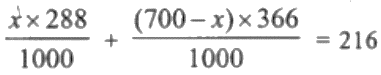288x + 235200 – 336x = 216000

288x – 336x = 216000 – 235200

x = -19200/-48 = 400

Therefore, the weight of almond = 400 gm and the weight of cashew = (700 – 400) gm = 300 gm

2. Drawing pencils cost 80 paise each and coloured pencils cost Rs. 1.10 each. If altogether two dozen pencils cost Rs. 21.60, how many coloured pencils are there?

Solution:

Let the number of drawing pencils be x

And let the number of coloured pencils be y

Then, according to the given conditions, we have

x + y = 2 x 12 [1 dozen = 12]

x + y = 24 … (i)

And,

x × 80/100 + y x 1.10 = 21.60 [As 80 paise = Rs 80/100]

80x/100 + 1.10y = 21.60

(80x + 110y)/100 = 21.60

80x + 110y = 2160

8x + 11y = 216 … (ii)

Performing 8 x (i) – (ii), we have

8x + 8y = 192

8x + 11y = 216

(-)–(-)—-(-)—–

-3y = -24

y = -24/-3

y = 8

On substituting the value of y in equation (i), we get

x + 8 = 24

x = 24 – 8

x = 16

Therefore, the number of coloured pencils is y = 8

3. Shikha works in a factory. In one week, she earned Rs. 390 for working 47 hours, of which 7 hours were overtime. The next week she earned Rs. 416 for working 50 hours, of which 8 hours were overtime. What is Shikha’s hourly earning rate?

Solution:

Let’s assume the earning’s of Shikha be Rs x per regular hour and Rs y per hour during overtime.

Then according to the conditions given in the problem, we have

40x + 7y = 390 … (i)

And,

42x + 8y = 416 … (ii)

Performing 8 x (i) – 7 x (ii), we get

320x + 56y = 3120

294x + 56y = 2912

(-)—-(-)——(-)—–

26x = 208

x = 208/26

On substituting the value of x in equation (i), we have

40 x 8 + 7y = 390

320 + 7y = 390

7y = 390 – 320

7y = 70

y = 70/7 = 10

Therefore, shikha’s earning is Rs 8 per regular hour and Rs 10 per hour overetime.

4. The sum of the digits of a two digit number is 7. If the digits are reversed, the new number increased by 3 equals 4 times the original number. Find the number.

Solution:

Let’s take the digit at tens place = x

And let the digit at unit place = y

So, the number = 10 × x + 1 × y = 10x + y

Reversing the number = 10 × y + 1 × x = 10y + x

Now, according to the conditions given in the problem, we have

x + y = 7… (i)

And,

10y + x = 4(10x + y) – 3

10y + x = 40x + 4y – 3

40x – x + 4y – 10y = 3

39x – 6y = 3

13x – 2y = 1 … (ii)

Performing 2 x (i) + (ii) to solve, we have

2x +2 y = 14

13x – 2y = 1

——————

15x = 15

x = 15/15

x = 1

On substituting the value of x in equation (i), we have

1 + y = 7

y = 7 – 1

y = 8

Therefore, the number is 10x + y = 10(1) + 8 = 18.

5. Three years hence a man’s age will be three times his son’s age and 7 years ago he was seven times as old as his son. How old are they now?

Solution:

Let the present age of man be x years

And his son’s present age be y years

So, three years hence the man’s age will be (x + 3) years

And his son’s age 3 years hence will be (y + 3) years

Now, 7 years ago the age of the man will be (x – 7) years

And the age of his son 7 years ago will be (y – 7) years

Then according to first given condition, we have

(x + 3) = 3 (y + 3)

x + 3 = 3y + 9

x – 3y = 9 – 3

x – 3y = 6 … (i)

Next, according to second given condition, we have

(x – 7) = 7(y – 7)

x – 7 = 7y – 49

x – 7y = -49 + 7

x – 7y = -42 … (ii)

Subtracting (i) from (ii), we get

x – 7y = -42

x – 3y = 6

(-)—(+)—(-)—-

-4y = -48

y = 48/4

y = 12

On substituting the value of x in equation (i), we have

x – 3(12) = 6

x – 36 = 6

x = 6 + 36

x = 42

Therefore, the man’s age is 42 years and his son’s age is 12 years.

6. Rectangles are drawn on line segments of fixed lengths. When the breadths are 6 m and 5 m respectively the sum of the areas of the rectangles is 83 m². But if the breadths are 5 m and 4 m respectively the sum of the areas is 68 m². Find the sum of the areas of the squares drawn on the line segments.

Solution:

Let the length of first fixed line segment be x

And the length of second line segment be y

In first case, when the breadths are 6 m and 5m the sum of the areas = 83 m2

⇒ 6x + 5y = 83 … (i)

And, in the second case when breadths are 5 m and 4 m the sum of areas = 68 m2

⇒ 5x + 4y = 68 … (ii)

Performing 4 x (i) – 5 x (ii) to solve, we get

24x + 20y = 332

25x + 20y = 340

(-)—-(-)——(-)—-

-x = -8

x = 8

On substituting the value of x in equation (i), we get

6(8) + 5y = 83

48 + 5y = 83

5y = 83 – 48

5y = 35

y = 35/7

y = 5

Therefore, the first line segment’s length is 8 m and the second line segment’s length is 7 m.

And the sum of areas of the squares on these two line segments = 82 + 72 = 64 + 49 = 113 m2.

7. Rectangles are drawn on line segments of fixed lengths. When the breadths are 6 m and 5 m respectively the sum of the areas of the rectangles is 83 m². But if the breadths are 5 m and 4 m respectively the sum of the areas is 68 m². Find the sum of the areas of the squares drawn on the line segments.

Solution:

Let’s consider the length of room as x metre

And the breadth of the room = y metre

So, the area of the room = length x breadth = xy sq. metre

Now, according to the first condition given in the problem, we have

xy = (x + 1) (y + 1) + 1 – 21

xy = xy + x + y + 1 – 21

x + y = 20 … (i)

And, according to the second condition, we have

xy = (x – 1) (y + 2) – 14

xy = xy – y + 2x – 2 – 14

2x – y = 16 … (ii)

Adding (i) and (ii), we get

x + y = 20

2x – y = 16

—————–

3x = 36

x = 36/3

x = 12

On substituting the value of x in equation (i), we get

12 + y = 20

y = 20 – 12

y = 8

Thus, the length of the room = 12 m and the breadth of the room = 8 m

Therefore, the perimeter of the room = 2 x (length + breadth)

= 2 x (12 + 8) = 2 x 20

= 40 m

8. The lengths (in metres) of the sides of a triangle are 2x + y/2, 5x/3 + y + ½ and 2x/3 + 2y + 5/2. If the triangle is equilateral, find the its perimeter.

Solution:

Given, the length of the sides of an equilateral triangle are 2x + y/2, 5x/3 + y + ½ and 2x/3 + 2y + 5/2.

Perimeter = (2x + y/2) + (5x/3 + y + ½) + (2x/3 + 2y + 5/2)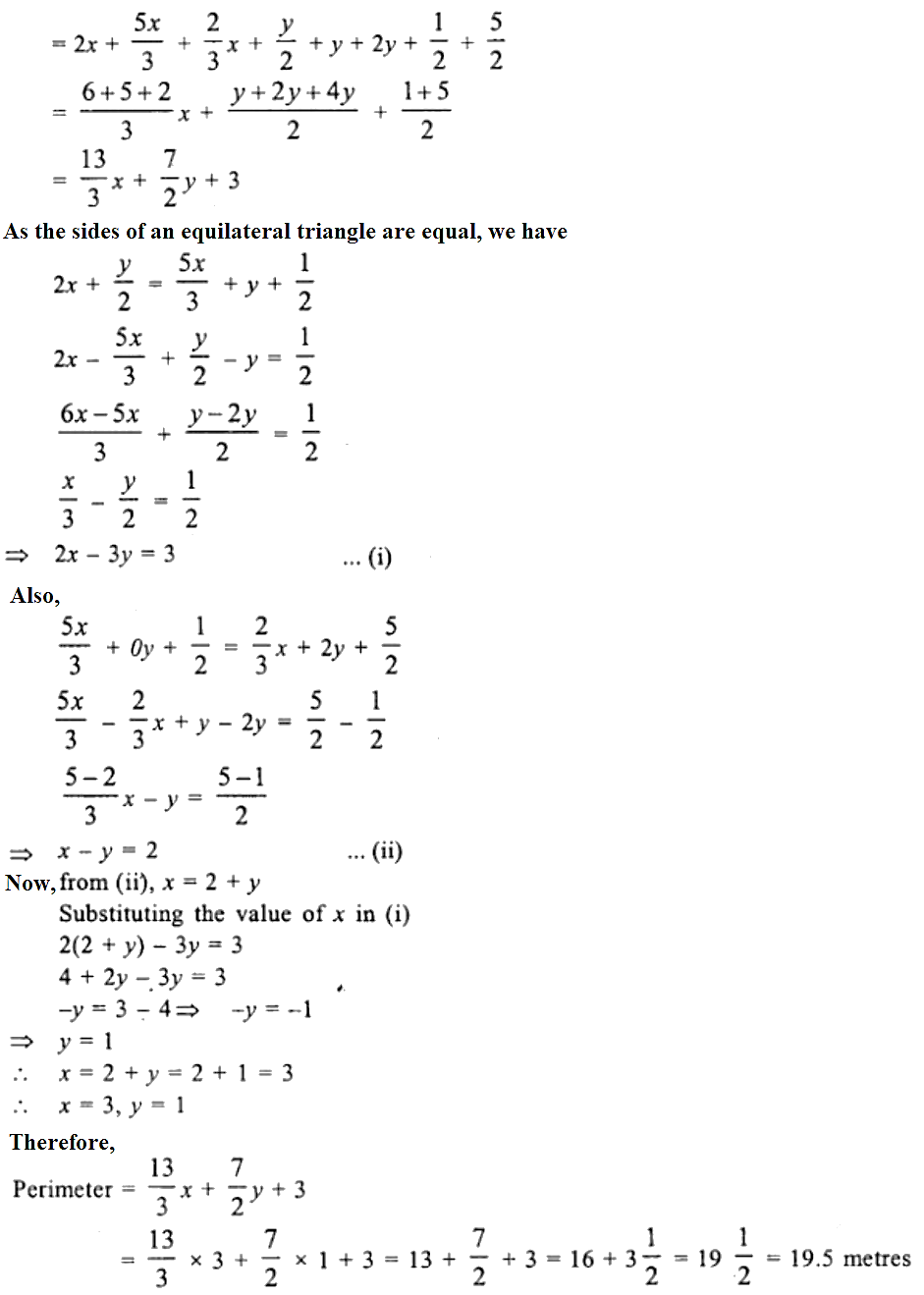9. On Diwali eve, two candles, one of which is 3 cm longer than the other are lighted. The longer one is lighted at 530 p.m. and the shorter at 7 p.m. At 930 p.m. they both are of the same length. The longer one burns out at 1130 p.m. and the shorter one at 11 p.m. How long was each candle originally?

Solution:

Let’s assume that the longer candle shorten at the rate of x cm/hr in burning case and the smaller candle shorter at the rate of y cm/hr.

Given, in burning case the longer candle burns out completely in 6 hours and that the smaller candle in 4 hours,

So, their lengths are 6x cm and 4y cm respectively.

Then, according to the first condition given in the problem, we have

6x = 4y + 3

6x – 4y = 3 … (i)

At 9:30 p.m the length of longer candle = (6x – 4x) cm = 2x cm

At 9:30 p.m the length of smaller candle = (4y – 5y/2) cm = (8y – 5y)/2 cm = 3y/2 cm

Now, according to the second condition given in the problem, we have

2x = 3y/2 [Since both the candles have same length at 9:30 p.m.]

4x = 3y

4x – 3y = 0 … (ii)

Performing 3 x (i) – 4 x (ii), we have

18x – 12y = 9

16x – 12y = 0

(-)–(+)—–(-)—

2x = 9

x = 9/2 = 4.5 cm/ hr

On substituting the value of x in equation (2), we get

4(4.5) – 3y = 0

18 – 3y = 0

3y = 18

y = 18/3

y = 6 cm/ hr

Therefore,

Length of longer candle = 6x cm = 6 x 4.5 cm = 27 cm

Length of smaller candle = 4y cm = 4 x 6 cm = 24 cm